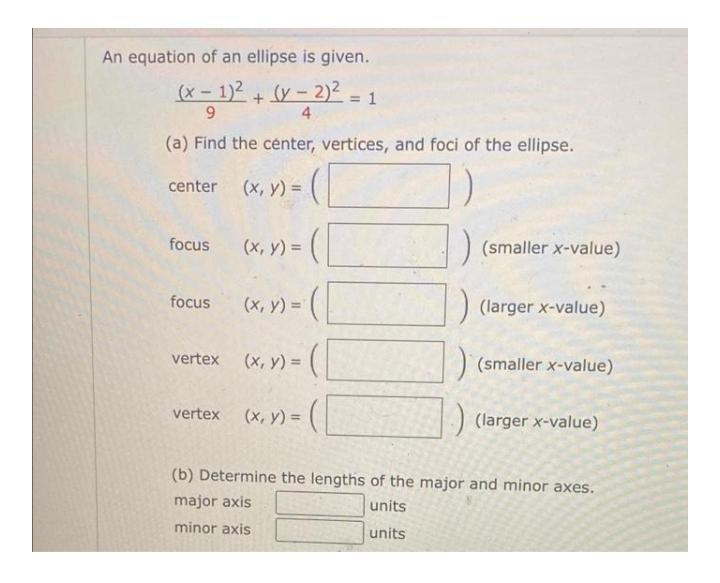Question:

# An equation of an ellipse is given. (x - 1)2 + (y - 2) 9 4 (a) Find the center, vertices, and foci of the ellipse. (132 = 2 = 1An equation of an ellipse is given. (x - 1)2 + (y - 2) 9 4 (a) Find the center, vertices, and foci of the ellipse. (132 = 2 = 1 center (x, y) = focus (x, y) = (smaller x-value) focus (larger x-value) (x, y) =( vertex (x, y) =( ) (smaller x-value) ) :) (larger x-value) vertex (x,y) = (b) Determine the lengths of the major and minor axes. major axis units minor axis units